# ML Aggarwal Solutions for Class 7 Maths Chapter 6 Ratio and Proportion

ML Aggarwal Solutions for Class 7 Maths Chapter 6 Ratio and Proportion help students to understand the concepts precisely and also develop a strong base in the subject. The solutions improve problem solving skills of students, which is very important in achieving high marks in academics. This also helps them pursue their desired course in further studies. Here, students can download ML Aggarwal Solutions for Class 7 Maths Chapter 6 Ratio and Proportion PDF, from the links which are mentioned below

This chapter contains problems on determining the ratio and proportion of various word problems. Students are suggested to practice these solutions repeatedly to ace the exam with ease. The solutions provide thorough explanation of each and every concept covered here, based on the latest syllabus of the prescribed board.

## ML Aggarwal Solutions for Class 7 Maths Chapter 6 Ratio and Proportion Download PDF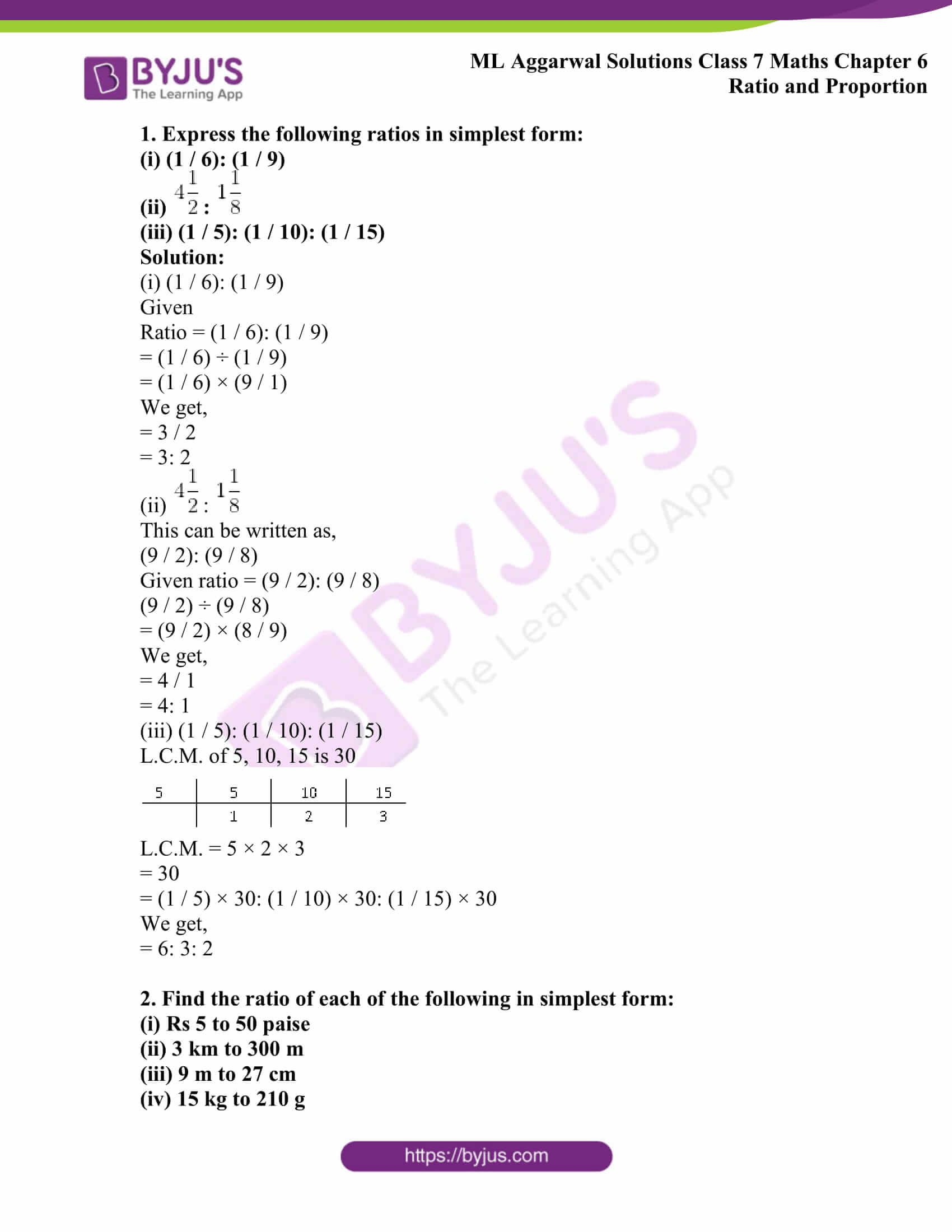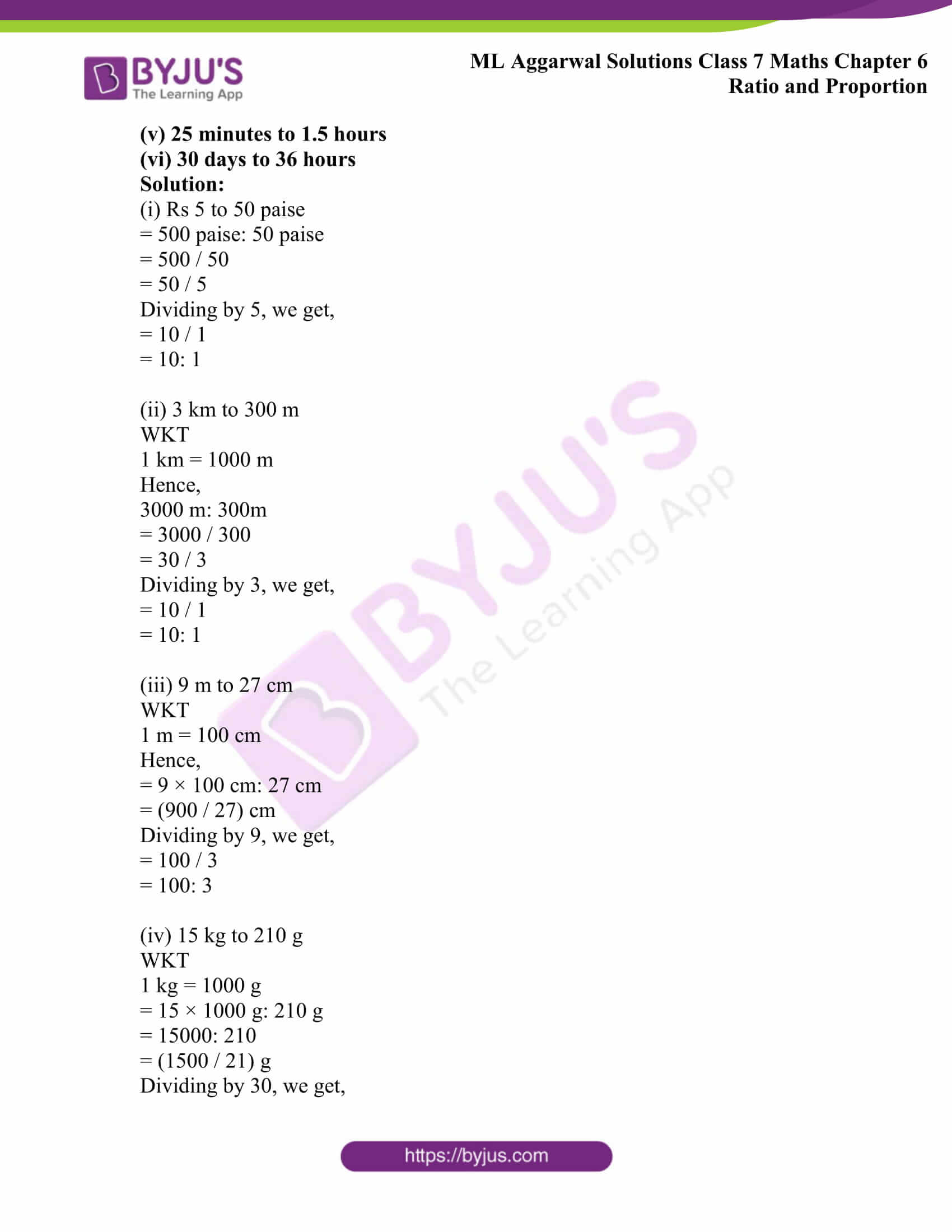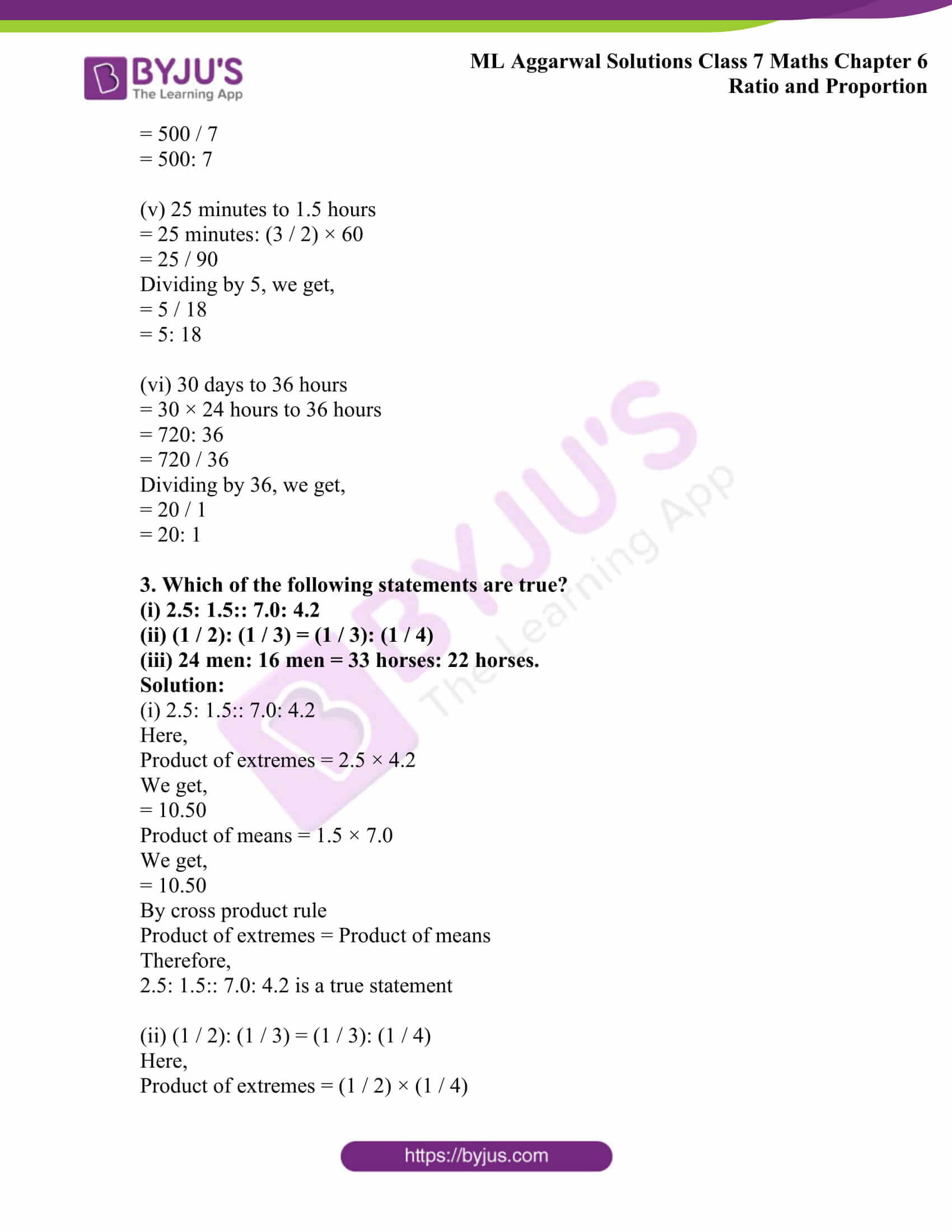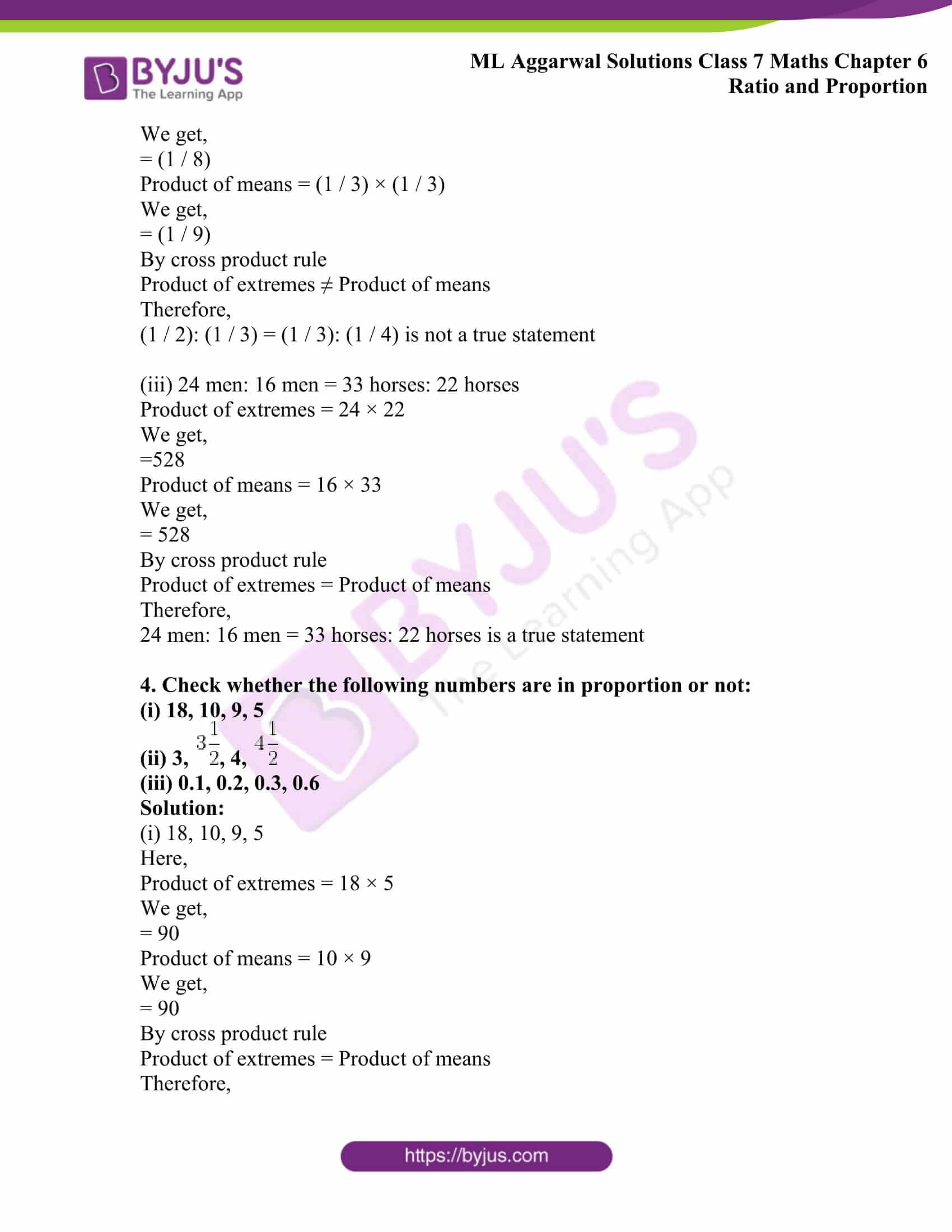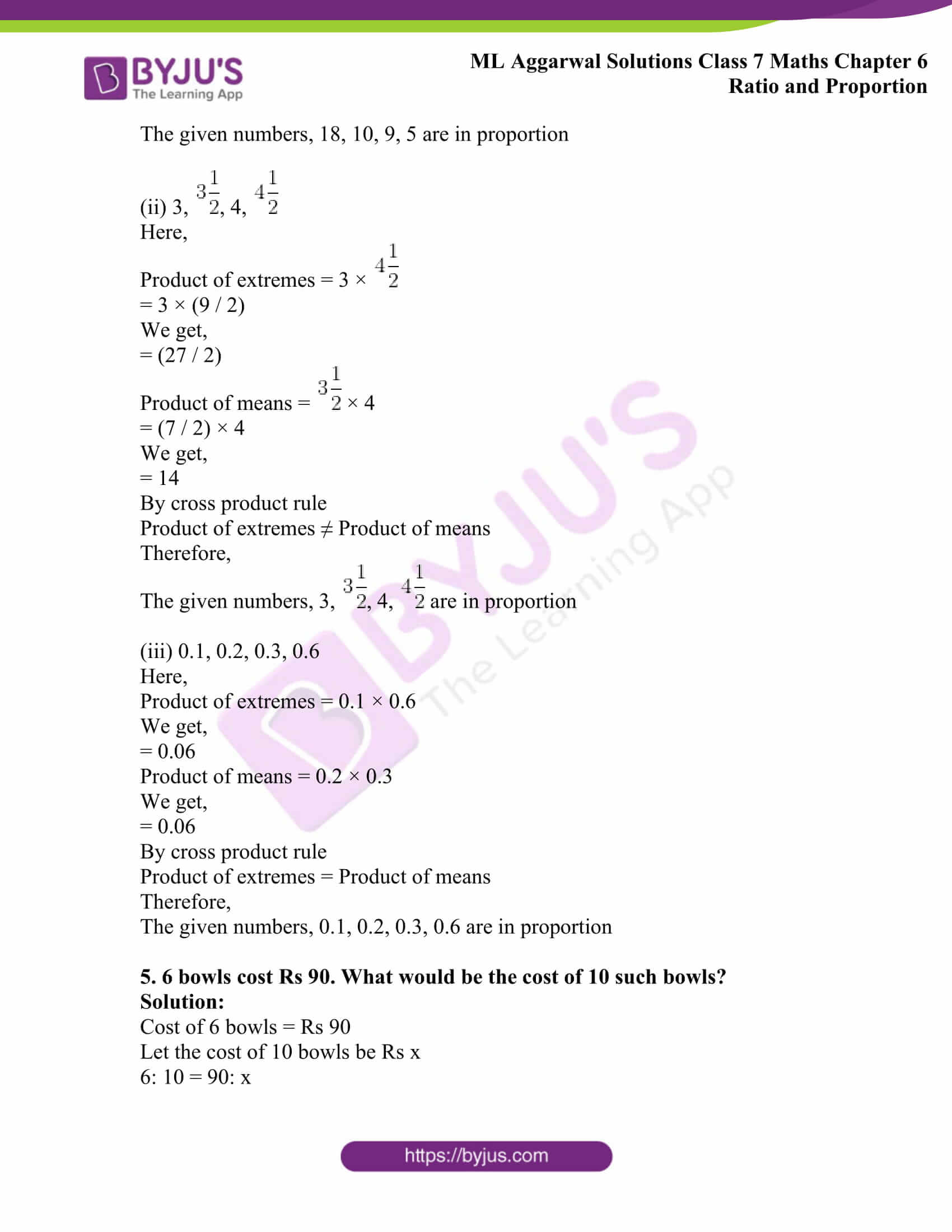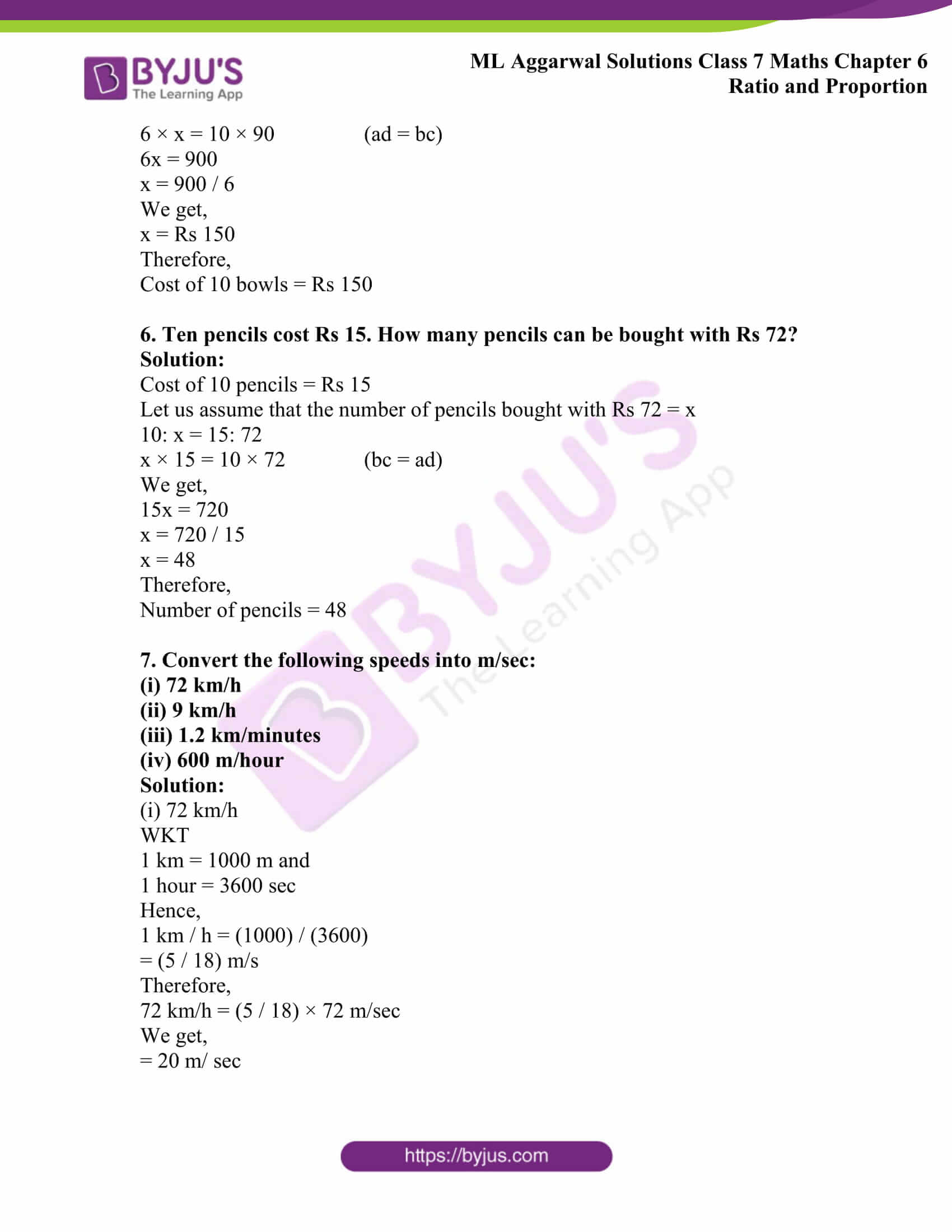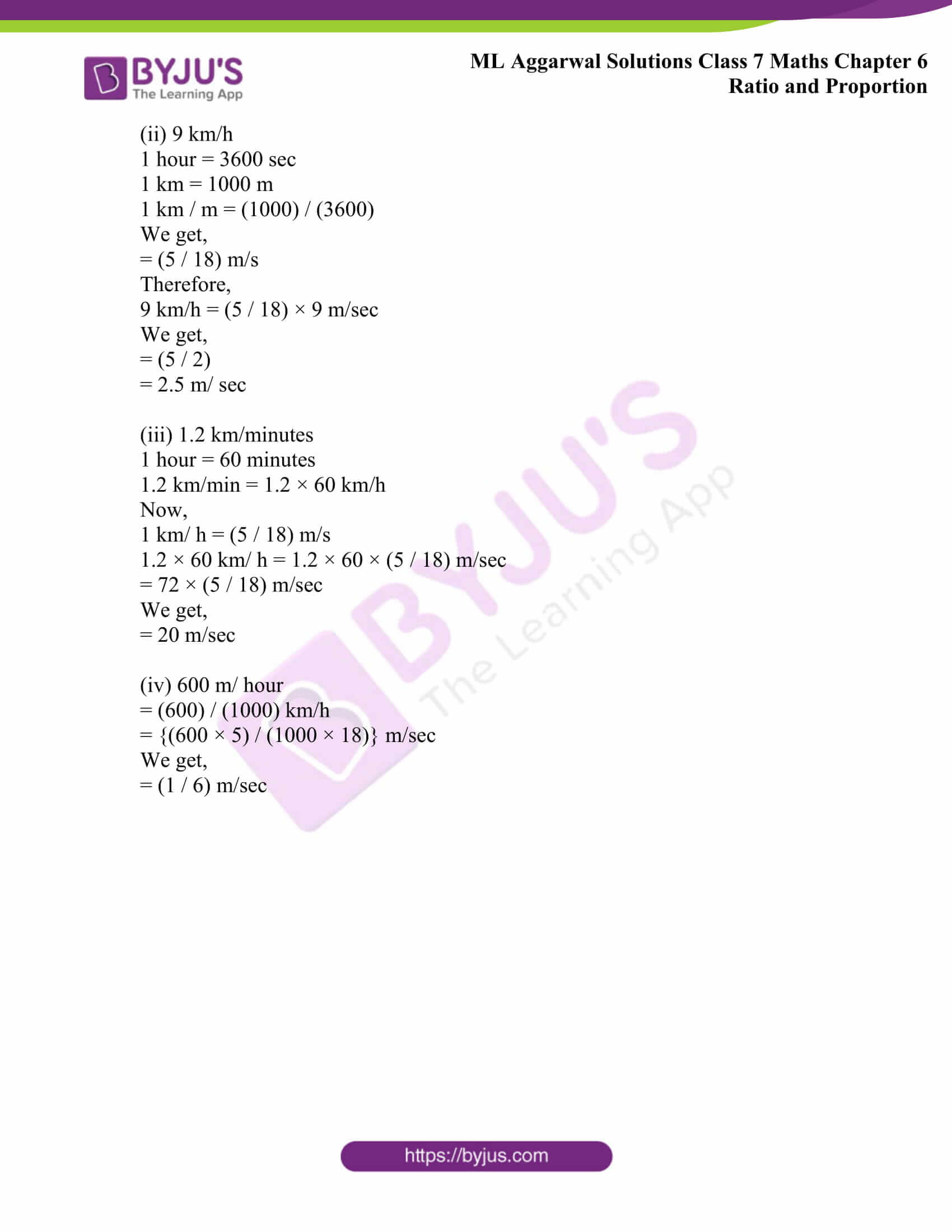## Access ML Aggarwal Solutions for Class 7 Maths Chapter 6 Ratio and Proportion

1. Express the following ratios in simplest form:

(i) (1 / 6): (1 / 9)

(ii)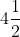: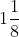(iii) (1 / 5): (1 / 10): (1 / 15)

Solution:

(i) (1 / 6): (1 / 9)

Given

Ratio = (1 / 6): (1 / 9)

= (1 / 6) ÷ (1 / 9)

= (1 / 6) × (9 / 1)

We get,

= 3 / 2

= 3: 2

(ii):This can be written as,

(9 / 2): (9 / 8)

Given ratio = (9 / 2): (9 / 8)

(9 / 2) ÷ (9 / 8)

= (9 / 2) × (8 / 9)

We get,

= 4 / 1

= 4: 1

(iii) (1 / 5): (1 / 10): (1 / 15)

L.C.M. of 5, 10, 15 is 30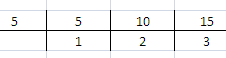L.C.M. = 5 × 2 × 3

= 30

= (1 / 5) × 30: (1 / 10) × 30: (1 / 15) × 30

We get,

= 6: 3: 2

2. Find the ratio of each of the following in simplest form:

(i) Rs 5 to 50 paise

(ii) 3 km to 300 m

(iii) 9 m to 27 cm

(iv) 15 kg to 210 g

(v) 25 minutes to 1.5 hours

(vi) 30 days to 36 hours

Solution:

(i) Rs 5 to 50 paise

= 500 paise: 50 paise

= 500 / 50

= 50 / 5

Dividing by 5, we get,

= 10 / 1

= 10: 1

(ii) 3 km to 300 m

WKT

1 km = 1000 m

Hence,

3000 m: 300m

= 3000 / 300

= 30 / 3

Dividing by 3, we get,

= 10 / 1

= 10: 1

(iii) 9 m to 27 cm

WKT

1 m = 100 cm

Hence,

= 9 × 100 cm: 27 cm

= (900 / 27) cm

Dividing by 9, we get,

= 100 / 3

= 100: 3

(iv) 15 kg to 210 g

WKT

1 kg = 1000 g

= 15 × 1000 g: 210 g

= 15000: 210

= (1500 / 21) g

Dividing by 30, we get,

= 500 / 7

= 500: 7

(v) 25 minutes to 1.5 hours

= 25 minutes: (3 / 2) × 60

= 25 / 90

Dividing by 5, we get,

= 5 / 18

= 5: 18

(vi) 30 days to 36 hours

= 30 × 24 hours to 36 hours

= 720: 36

= 720 / 36

Dividing by 36, we get,

= 20 / 1

= 20: 1

3. Which of the following statements are true?

(i) 2.5: 1.5:: 7.0: 4.2

(ii) (1 / 2): (1 / 3) = (1 / 3): (1 / 4)

(iii) 24 men: 16 men = 33 horses: 22 horses.

Solution:

(i) 2.5: 1.5:: 7.0: 4.2

Here,

Product of extremes = 2.5 × 4.2

We get,

= 10.50

Product of means = 1.5 × 7.0

We get,

= 10.50

By cross product rule

Product of extremes = Product of means

Therefore,

2.5: 1.5:: 7.0: 4.2 is a true statement

(ii) (1 / 2): (1 / 3) = (1 / 3): (1 / 4)

Here,

Product of extremes = (1 / 2) × (1 / 4)

We get,

= (1 / 8)

Product of means = (1 / 3) × (1 / 3)

We get,

= (1 / 9)

By cross product rule

Product of extremes ≠ Product of means

Therefore,

(1 / 2): (1 / 3) = (1 / 3): (1 / 4) is not a true statement

(iii) 24 men: 16 men = 33 horses: 22 horses

Product of extremes = 24 × 22

We get,

=528

Product of means = 16 × 33

We get,

= 528

By cross product rule

Product of extremes = Product of means

Therefore,

24 men: 16 men = 33 horses: 22 horses is a true statement

4. Check whether the following numbers are in proportion or not:

(i) 18, 10, 9, 5

(ii) 3,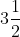, 4,(iii) 0.1, 0.2, 0.3, 0.6

Solution:

(i) 18, 10, 9, 5

Here,

Product of extremes = 18 × 5

We get,

= 90

Product of means = 10 × 9

We get,

= 90

By cross product rule

Product of extremes = Product of means

Therefore,

The given numbers, 18, 10, 9, 5 are in proportion

(ii) 3,, 4,Here,

Product of extremes = 3 ×= 3 × (9 / 2)

We get,

= (27 / 2)

Product of means =× 4

= (7 / 2) × 4

We get,

= 14

By cross product rule

Product of extremes ≠ Product of means

Therefore,

The given numbers, 3,, 4,are in proportion

(iii) 0.1, 0.2, 0.3, 0.6

Here,

Product of extremes = 0.1 × 0.6

We get,

= 0.06

Product of means = 0.2 × 0.3

We get,

= 0.06

By cross product rule

Product of extremes = Product of means

Therefore,

The given numbers, 0.1, 0.2, 0.3, 0.6 are in proportion

5. 6 bowls cost Rs 90. What would be the cost of 10 such bowls?

Solution:

Cost of 6 bowls = Rs 90

Let the cost of 10 bowls be Rs x

6: 10 = 90: x

6 × x = 10 × 90 (ad = bc)

6x = 900

x = 900 / 6

We get,

x = Rs 150

Therefore,

Cost of 10 bowls = Rs 150

6. Ten pencils cost Rs 15. How many pencils can be bought with Rs 72?

Solution:

Cost of 10 pencils = Rs 15

Let us assume that the number of pencils bought with Rs 72 = x

10: x = 15: 72

x × 15 = 10 × 72 (bc = ad)

We get,

15x = 720

x = 720 / 15

x = 48

Therefore,

Number of pencils = 48

7. Convert the following speeds into m/sec:

(i) 72 km/h

(ii) 9 km/h

(iii) 1.2 km/minutes

(iv) 600 m/hour

Solution:

(i) 72 km/h

WKT

1 km = 1000 m and

1 hour = 3600 sec

Hence,

1 km / h = (1000) / (3600)

= (5 / 18) m/s

Therefore,

72 km/h = (5 / 18) × 72 m/sec

We get,

= 20 m/ sec

(ii) 9 km/h

1 hour = 3600 sec

1 km = 1000 m

1 km / m = (1000) / (3600)

We get,

= (5 / 18) m/s

Therefore,

9 km/h = (5 / 18) × 9 m/sec

We get,

= (5 / 2)

= 2.5 m/ sec

(iii) 1.2 km/minutes

1 hour = 60 minutes

1.2 km/min = 1.2 × 60 km/h

Now,

1 km/ h = (5 / 18) m/s

1.2 × 60 km/ h = 1.2 × 60 × (5 / 18) m/sec

= 72 × (5 / 18) m/sec

We get,

= 20 m/sec

(iv) 600 m/ hour

= (600) / (1000) km/h

= {(600 × 5) / (1000 × 18)} m/sec

We get,

= (1 / 6) m/sec